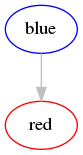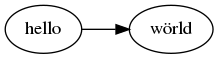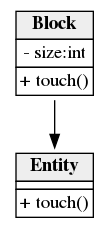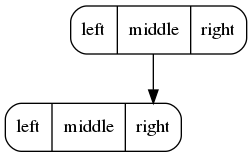## graphp /graphviz

GraphViz graph drawing for the mathematical graph/network library GraPHP.

##### Details

Homepage

Source

Issues

965 121

67

16

0

242

10

36

v0.2.2 2019-10-04 13:30 UTC

This package is auto-updated.

Last update: 2023-05-27 11:19:08 UTCGraphViz graph drawing for the mathematical graph/network library GraPHP.

Development version: This branch contains the code for the upcoming 1.0 release. For the code of the current stable 0.2 release, check out the `0.2.x` branch.

The upcoming 1.0 release will be the way forward for this package. However, we will still actively support 0.2.x for those not yet on the latest version. See also installation instructions for more details.

The library supports visualizing graph images, including them into webpages, opening up images from within CLI applications and exporting them as PNG, JPEG or SVG file formats (among many others). Because graph drawing is a complex area on its own, the actual layouting of the graph is left up to the excelent GraphViz "Graph Visualization Software" and we merely provide some convenient APIs to interface with GraphViz.

## Quickstart examples

Once installed, let's build and display a sample graph:

```\$graph = new Graphp\Graph\Graph();

\$blue = \$graph->createVertex();
\$blue->setAttribute('id', 'blue');
\$blue->setAttribute('graphviz.color', 'blue');

\$red = \$graph->createVertex();
\$red->setAttribute('id', 'red');
\$red->setAttribute('graphviz.color', 'red');

\$edge = \$graph->createEdgeDirected(\$blue, \$red);
\$edge->setAttribute('graphviz.color', 'grey');

\$graphviz = new Graphp\GraphViz\GraphViz();
\$graphviz->display(\$graph);```

The above code will open your default image viewer with the following image:## Attributes

GraphViz supports a number of attributes on the graph instance itself, each vertex instance (GraphViz calls these "nodes") and edge instance. Any of these GraphViz attributes are supported by this library and have to be assigned using GraPHP attributes as documented below.

For the full list of all GraphViz attributes, please refer to the GraphViz documentation.

Note that all attributes use UTF-8 encoding (Unicode) and will be quoted and escaped by default, so a `ö` and `>` will appear as-is and will not be interpreted as HTML. See also HTML-like labels below for more details.

### Graph attributes

GraphViz supports a number of attributes on the graph instance itself. Any of these GraphViz attributes are supported by this library and have to be assigned on the graph instance with the `graphviz.graph.` prefix like this:

```\$graph = new Graphp\Graph\Graph();
\$graph->setAttribute('graphviz.graph.bgcolor', 'transparent');```

Note how this uses the `graphviz.graph.` prefix and not just `graphviz.`. This is done for consistency reasons with respect to default vertex and edge attributes as documented below.

For example, the `rankdir` attribute can be used to change the orientation to horizontal mode (left to right) like this:

```\$graph = new Graphp\Graph\Graph();
\$graph->setAttribute('graphviz.graph.rankdir', 'LR');

\$hello = \$graph->createVertex();
\$hello->setAttribute('id', 'hello');
\$world = \$graph->createVertex();
\$world->setAttribute('id', 'wörld');
\$graph->createEdgeDirected(\$hello, \$world);```Additionally, this library accepts an optional `graphviz.name` attribute that will be used as the name (or ID) for the root graph object in the DOT output if given. Unless explicitly assigned, this will be omitted by default. It is common to assign a `G` here, but usually there should be no need to assign this. Among others, this may be used as the title or tooltip in SVG output.

```\$graph = new Graphp\Graph\Graph();
\$graph->setAttribute('graphviz.name', 'G');

\$graph->createVertex();```

### Vertex attributes

GraphViz supports a number of attributes on each vertex instance (GraphViz calls these "node" attributes). Any of these GraphViz attributes are supported by this library and have to be assigned on the respective vertex instance with the `graphviz.` prefix like this:

```\$graph = new Graphp\Graph\Graph();

\$blue = \$graph->createVertex();
\$blue->setAttribute('graphviz.color', 'blue');```

Additionally, GraphViz also supports default attributes for all vertices. Any of these GraphViz attributes are supported by this library and have to be assigned on the graph instance with the `graphviz.node.` prefix like this:

```\$graph = new Graphp\Graph\Graph();
\$graph->setAttribute('graphviz.node.color', 'grey');

\$grey = \$graph->createVertex();```

These default attributes can be overriden on each vertex instance by explicitly assigning the same attribute on the respective vertex instance like this:

```\$graph = new Graphp\Graph\Graph();
\$graph->setAttribute('graphviz.node.color', 'grey');

\$blue = \$graph->createVertex();
\$blue->setAttribute('graphviz.color', 'blue');```

Note how this uses the `graphviz.node.` prefix and not `graphviz.vertex.`. This is done for consistency reasons with respect to how GraphViz assigns these default attributes in its DOT output.

### Edge attributes

GraphViz supports a number of attributes on each edge instance. Any of these GraphViz attributes are supported by this library and have to be assigned on the respective edge instance with the `graphviz.` prefix like this:

```\$graph = new Graphp\Graph\Graph();

\$a = \$graph->createVertex();
\$b = \$graph->createVertex();

\$blue = \$graph->createEdgeDirected(\$a, \$b);
\$blue->setAttribute('graphviz.color', 'blue');```

Additionally, GraphViz also supports default attributes for all edges. Any of these GraphViz attributes are supported by this library and have to be assigned on the graph instance with the `graphviz.edge.` prefix like this:

```\$graph = new Graphp\Graph\Graph();
\$graph->setAttribute('graphviz.edge.color', 'grey');

\$a = \$graph->createVertex();
\$b = \$graph->createVertex();

\$grey = \$graph->createEdgeDirected(\$a, \$b);```

These default attributes can be overriden on each edge instance by explicitly assigning the same attribute on the respective edge instance like this:

```\$graph = new Graphp\Graph\Graph();
\$graph->setAttribute('graphviz.edge.color', 'grey');

\$a = \$graph->createVertex();
\$b = \$graph->createVertex();

\$blue = \$graph->createEdgeDirected(\$a, \$b);
\$blue->setAttribute('graphviz.color', 'blue');```

## Labels

### Vertex labels

By default, GraphViz will always render the vertex ID as the label. If you do not assign an explicit `id` attribute to a vertex, this library will automatically assign a vertex ID starting at `1` in the DOT output and GraphViz will automatically render this vertex ID as the label. The following example will automatically assign `1` and `2` as the label:

```\$graph = new Graphp\Graph\Graph();

\$v1 = \$graph->createVertex();
\$v2 = \$graph->createVertex();```

If you assign an `id` attribute to a vertex, this library will automatically use it as the vertex ID in the DOT output and GraphViz will automatically render this vertex ID as the label. The following example will automatically assign `blue` as the label:

```\$graph = new Graphp\Graph\Graph();

\$a = \$graph->createVertex();
\$a->setAttribute('id', 'blue');```

If you assign a `balance` attribute to a vertex, this library will automatically include a `label` attribute that appends the balance value in parenthesis. The following example will automatically assign `blue (+10)` as the label:

```\$graph = new Graphp\Graph\Graph();

\$blue = \$graph->createVertex();
\$blue->setAttribute('id', 'blue');
\$blue->setAttribute('balance', 10);```

You can use vertex attributes to explicitly assign a custom `label` attribute. Note that any balance value will still be appended like in the previous example.

```\$graph = new Graphp\Graph\Graph();

\$blue = \$graph->createVertex();
\$blue->setAttribute('id', 'blue');
\$blue->setAttribute('graphviz.label', 'Hello world!');```

Note that all attributes will be quoted and escaped by default, so a `>` will appear as-is and will not be interpreted as HTML. See also HTML-like labels below for more details.

Also note that you should either define no vertex IDs at all or all vertex IDs. If you only define some vertex IDs, the automatic numbering may yield a vertex ID that is already used explicitly and overwrite some of its settings.

### Edge labels

By default, GraphViz will not render any label on an edge:

```\$graph = new Graphp\Graph\Graph();

\$a = \$graph->createVertex();
\$b = \$graph->createVertex();

\$edge = \$graph->createEdgeDirected(\$a, \$b);```

If you assign a `flow`, `capacity` or `weight` attribute to an edge, this library will automatically include a `label` attribute that includes these values. The following example will automatically assign `100` as the label for the weighted edge:

```\$graph = new Graphp\Graph\Graph();

\$a = \$graph->createVertex();
\$b = \$graph->createVertex();

\$edge = \$graph->createEdgeDirected(\$a, \$b);
\$edge->setAttribute('weight', 100);```

The following example will automatically assign `4/10` as the label for an edge with both flow and maximum capacity set:

```\$graph = new Graphp\Graph\Graph();

\$a = \$graph->createVertex();
\$b = \$graph->createVertex();

\$edge = \$graph->createEdgeDirected(\$a, \$b);
\$edge->setAttribute('flow', 4);
\$edge->setAttribute('capacity', 10);```

The following example will automatically assign `4/∞/100` as the label for a weighted edge with a flow and unlimited capacity:

```\$graph = new Graphp\Graph\Graph();

\$a = \$graph->createVertex();
\$b = \$graph->createVertex();

\$edge = \$graph->createEdgeDirected(\$a, \$b);
\$edge->setAttribute('flow', 4);
\$edge->setAttribute('capacity', null);
\$edge->setAttribute('weight', 100);```

You can use edge attributes to explicitly assign any custom `label` attribute. Note that any flow, capacity or weight value will still be appended like in the previous examples.

```\$graph = new Graphp\Graph\Graph();

\$a = \$graph->createVertex();
\$b = \$graph->createVertex();

\$edge = \$graph->createEdgeDirected(\$a, \$b);
\$edge->setAttribute('graphviz.label', 'important');```

### HTML-like labels

Note that all attributes will be quoted and escaped by default, so a `>` will appear as-is and will not be interpreted as HTML. GraphViz also supports HTML-like labels that support a subset of HTML features.

GraphViz requires any HTML-like label to be wrapped in `<` and `>` and only supports a limited subset of HTML features as documented above. In order to prevent automatic quoting and escaping, all HTML-like attribute values need to be passed with a trailing `_html` in the attribute name like this:

```\$graph = new Graphp\Graph\Graph();

\$a = \$graph->createVertex();
\$a->setAttribute('id', 'Entity');
\$a->setAttribute('graphviz.shape', 'none');
\$a->setAttribute('graphviz.label_html', '
<table cellspacing="0" border="0" cellborder="1">
<tr><td bgcolor="#eeeeee"><b>\N</b></td></tr>
<tr><td></td></tr>
<tr><td>+ touch()</td></tr>
</table>');

\$b = \$graph->createVertex();
\$graph->createEdgeDirected(\$b, \$a);
\$b->setAttribute('id', 'Block');
\$b->setAttribute('graphviz.shape', 'none');
\$b->setAttribute('graphviz.label_html', '
<table cellspacing="0" border="0" cellborder="1">
<tr><td bgcolor="#eeeeee"><b>\N</b></td></tr>
<tr><td>- size:int</td></tr>
<tr><td>+ touch()</td></tr>
</table>');```### Record-based nodes

Note that all attributes will be quoted and escaped by default, so a `>` will appear as-is and will not be interpreted as HTML. Similar to the above HTML-like labels, GraphViz also supports simple record-based nodes using the `record` and `Mrecord` shape attributes and structured label attributes.

GraphViz requires any record-based node label to be quoted, but uses special syntax to mark record fields and optional port names. In order to prevent automatic quoting and escaping, all record-based attribute values need to be passed with a trailing `_record` in the attribute name like this:

```\$graph = new Graphp\Graph\Graph();

\$a = \$graph->createVertex();
\$a->setAttribute('graphviz.shape', 'Mrecord');
\$a->setAttribute('graphviz.label_record', '<f0> left |<middle> middle |<f2> right'));

\$b = \$graph->createVertex();
\$b->setAttribute('graphviz.shape', 'Mrecord');
\$b->setAttribute('graphviz.label_record', '<f0> left |<f1> middle |<right> right'));

// a:middle -> b:right
\$edge = \$graph->createEdgeDirected(\$a, \$b);
\$edge->setAttribute('graphviz.tailport', 'middle');## Install

The recommended way to install this library is through Composer. New to Composer?

Once released, this project will follow SemVer. At the moment, this will install the latest development version:

`composer require graphp/graphviz:^1@dev`

This project aims to run on any platform and thus does not require any PHP extensions and supports running on legacy PHP 5.3 through current PHP 7+. It's highly recommended to use PHP 7+ for this project.

The graph drawing feature is powered by the excellent GraphViz software. This means you'll have to install GraphViz (`dot` executable). The Graphviz homepage includes complete installation instructions for most common platforms, users of Debian/Ubuntu-based distributions may simply invoke:

`sudo apt install graphviz`

## Tests

To run the test suite, you first need to clone this repo and then install all dependencies through Composer:

`composer install`

To run the test suite, go to the project root and run:

`vendor/bin/phpunit`